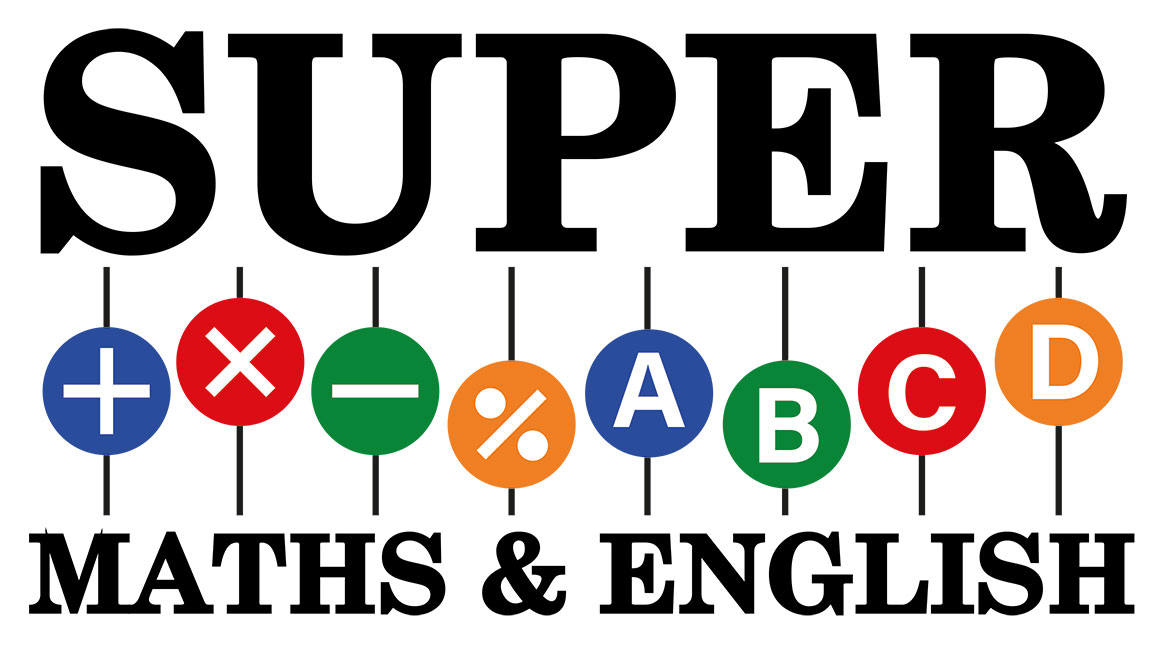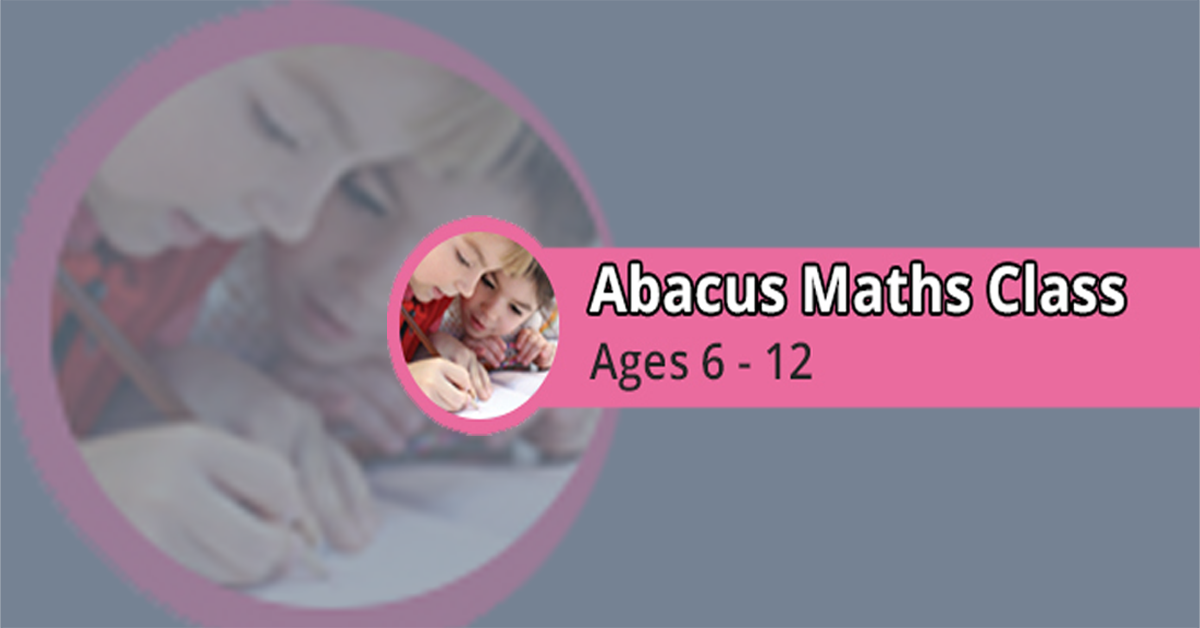Supermaths# Children’s Abacus Maths Classes

Childrens ABACUS Maths classes offer a friendly and educational introduction to the use of the Abacus tool and the underlying concepts. We then move onto using an abacus for basic addition and subtraction. Then we progress more throughout the program.

Here at Supermaths Academy we offer a child-friendly introduction to the use of the Abacus, both the tool and ultimately the mental theory concepts. We begin with finger theory, move onto an abacus tool before moving onto mental calculations. Each of our abacus levels lasts at least 12 weeks. This can differ depending on how well the pupil is coping with the current level.

There are two tracks of the course

1. Junior Track – Junior Track has 11 Levels.
2. Senior Track- Senior Track has 8 Levels.

### Level 1A

Abacus & Finger Theory

• 1 to 99 numbers

• +5 combination + 5 combinations and + 10 combinations

Mental Theory

• 1 & 2 digits (non combination)

### Level 2A

Abacus & Finger theory

• -10 combinations, +6 to +9 and -6 to -9 double combinations.

Abacus

• Multiplication 2 digits x 1 digit

• (with tables 1 to 9)

Mental theory

• 1 & 2 digits, +5 combination, -5 combination (+10 combinations)

### Level 3A

Abacus

• 2 & 3digits all combinations

• decimals 3 digits decimal addition and subtraction

• Multiplication 2 digits x 1digit, 3digits x 1 digit and 4 digit x1 digit.

Mental theory

• 2 digits all combinations

• Multiplication 2 digits x 1digit, 3digits x 1 digit

### Level 4A

Abacus

• 2,3 & 4 digits all combinations

• decimals 4 digits all combinations.

• Multiplication 3 & 4 digits x 1 digit, 2 digits x 2 digits.

• Division 2,3 and 4 digits ÷ 1 digits.</li

• Division 2 digits ÷1 digit

Mental theory

• 2 & 3 digits all combinations

• decimals 3 digits all combinations

• Multiplication 2,3 and 4 digits x 1 digit

### Level 5A

Abacus

• Addition & subtraction (3 & 4 digits all combinations)

• Multiplication (2x2digits, 3×2 digits)

• Division 3÷1 digits, 4÷1 digit, 3÷2 digits and 4÷2digits

Mental theory

• Addition & subtraction (2&3 digits all combinations)

• Decimal 4 digits all combinations

• Multiplication 3 x 1 digit,4×1 digit 2 x 2 digits

• Division 2÷1 digit, 3÷1digit, 4÷1digit

### Level 6A

Abacus

• Addition & subtraction (4 and 5 digits all combinations)

• Multiplication 4 x 2 digits

• Division 3÷2 digits, 4÷2 digits and 5÷2 digits

• Fractions Conversion

• BODMAS

Mental theory

• Addition & subtraction (2&3digits all combinations)

• Decimal 4 digits all combinations

• Multiplication 2x2digits, 3×2 digits

• Division 4÷1 digit, 3÷2

• Fractions conversion

### Level 7A

Abacus

• Addition & subtraction (4 and 5 digits all combinations)

• Decimal 5 digits all combinations

• Multiplication 3×3 digits, 4×2 digits

• Division 4÷2 digits, 5÷2 digits

• Fractions combined operations

• Percentage

Mental theory

• 2×3 digits all combinations

• decimal 5 digits all combinations

• Multiplication 3 x 2 digits

• Division 4÷1digits, 3÷2 digits

• Decimal multiplication

• Decimal divisions

• Factions

### Level 8A

Abacus

• 4 and 5 digits all combinations

• Division 5÷2 digits

• Square roots 3 and 4 digits combined operations

Mental theory

• Addition & subtraction (3 and 4 digits all combinations)

• Mixed calculations (÷, -, x, +) with bodmas rule

• decimal 5 digits all combinations

• Multiplication 3×2 digits

• Division 4÷1 digits, 3÷2 digits

• Decimal multiplication

• Decimal divisions

• Factions

As you can see we have a lot on offer for children to learn, and this will allow them to master their arithmetic skills. You can see videos of our students who are learning the abacus maths technique on our YouTube channel, this will allow you to see what is possible within a short length of time.

### Level One

Abacus & finger theory

• 1 to 99 numbers

• +5 combinations, -5 combinations (Test week 1 and Test Week 2)

• 2 Digits numbers

### Level Two

Abacus & finger theory

• 1 to 99 numbers

• +10 combinations (Test week 3 , Test Week 4 and Test Week 5)

• 2 Digits numbers

### Level Three

Abacus & finger theory

• 1 to 99 numbers

• -10 combinations(Test week 6 , Test Week 7 and Test Week 8)

• 2 Digits numbers

### Level Four

Abacus & finger theory

• 1 to 99 numbers

• +5 combination + 5 combinations and + 10 combinations

Mental theory

• 1 & 2 digits(non combination)

### Level Five

Abacus & Finger theory

• -10 combinations, +6 to +9 and -6 to -9 double combinations.

Abacus

• Multiplication 2 digits x 1 digit (with tables 1 to 9)

Mental theory

• 1 & 2 digits, +5 combination, -5 combination (+10 combinations)

### Level Six

Abacus

• 2 & 3digits all combinations

• Decimals 3 digits decimal addition and subtraction

• Multiplication 2 digits x 1digit, 3digits x 1 digit and 4 digit x1 digit.

Mental theory

• 2 digits all combinations

• Multiplication 2 digits x 1digit, 3digits x 1 digit

### Level Seven

Abacus

• 2,3 & 4 digits all combinations

• decimals 4 digits all combinations.

• Multiplication 3 & 4 digits x 1 digit, 2 digits x 2 digits.

• Division 2,3 and 4 digits ÷ 1 digits.

• Division 2 digits ÷1 digit

Mental theory

• 2 & 3 digits all combinations

• Decimals 3 digits all combinations

• Multiplication 2,3 and 4 digits x 1 digit,

### Level Eight

Abacus

• Addition & subtraction ( 3 & 4 digits all combinations )

• Multiplication ( 2×2 digits, 3×2 digits )

• Division 3÷1 digits, 4÷1 digit, 3÷2 digits and 4÷2 digits

Mental theory

• Addition & subtraction ( 2&3 digits all combinations )

• Decimal 4 digits all combinations

• Multiplication 3 x 1 digit, 4×1 digit 2 x 2 digits

• Division 2÷1 digit, 3÷1 digit, 4÷1 digit

### Level Nine

Abacus

• Addition & subtraction( 4 and 5 digits all combinations )

• Multiplication 4 x 2 digits

• Division 3÷2 digits,   4÷2 digits and 5÷2 digits

• Fractions Conversion

• BODMAS

Mental theory

• 2&3 digits all combinations

• Decimal 4 digits all combinations

• Multiplication 2x2digits, 3×2 digits

• Division 4÷1 digit, 3÷2 Fractions conversion, simple addition and subtraction

### Level Ten

Abacus

• Addition & subtraction ( 4 and 5 digits all combinations ) Decimal 5 digits all combinations

• Multiplication 3×3 digits, 4×2 digits

• Division 4÷2 digits, 5÷2 digits

• Fractions combined operations

• Percentage

Mental theory

• Addition & subtraction ( 2×3 digits all combinations )

• Decimal 5 digits all combinations

• Multiplication 3 x 2 digits

• Division 4÷1 digits, 3÷2 digits

• Decimal multiplication

• Decimal divisions

• Factions

### Level Eleven

Abacus

• Addition & subtraction ( 4 and 5 digits all combinations )

• Division 5÷2 digits

• Square roots 3 and 4 digits combined operations

Mental theory

• Addition & subtraction ( 3 and 4 digits all combinations )

• Mixed calculations (÷, -, x, +) with BODMAS rule

• Decimal 5 digits all combinations

• Multiplication 3×2 digits

• Division 4÷1 digits, 3÷2 digits